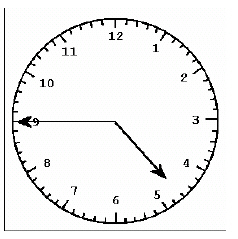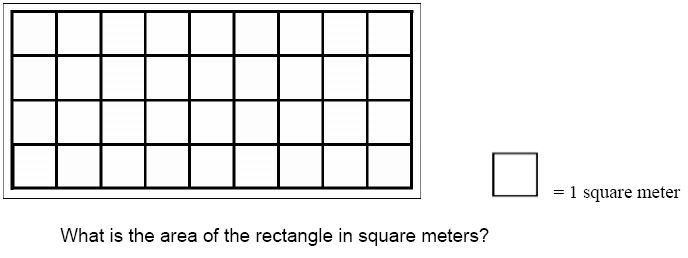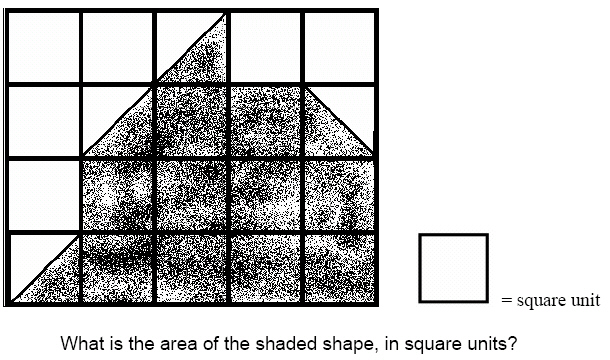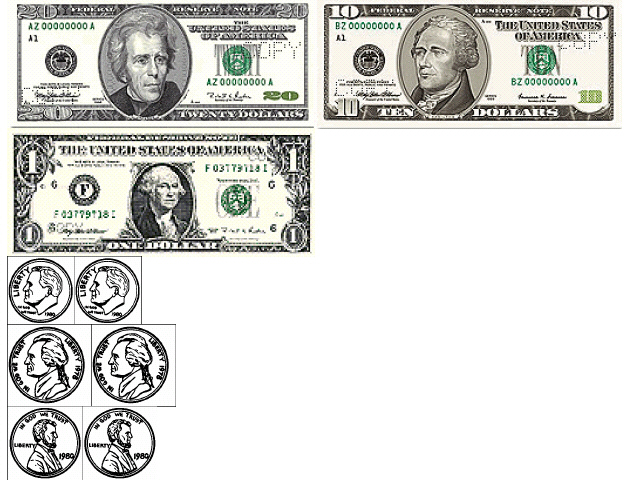Name:    2nd Grade Math Proficient Test 7

Multiple Choice
Identify the choice that best completes the statement or answers the question.

1.

Measurement – Time, Temperature – RIT 181 – 190What time is it?
 a. 5:10 d. 6:13 b. 4:12 e. 5:12 c. 2:28

2.

6 p.m. is:
 a. 4 hours after 2 p.m. d. 6 hours after midnight b. 4 hours after 10 p.m. e. 4 hours before midnight c. 6 hours before noon

3.

The time 30 minutes after 5:15 is:
 a. 5:18 d. 6:15 b. 5:45 e. 5:15 c. 4:45

4.

What time is 25 minutes later than the time on the clock?a. 5:15 d. 4:45 b. 4:10 e. 5:05 c. 5:10

5.

Measurement – Area, Perimeter, Circumference – RIT 191 – 200a. 9 d. 13 b. 32 e. 50 c. 36

6.a. 12 d. 30 b. 10 e. 16 c. 14

7.a. 11 inches d. 21 inches b. 16 inches e. 22 inches c. 14 inches

8.

Measurement – Length, Weight, Volume – RIT 191 – 200
How long is the pencil?a. 45 cm. d. 13 cm. b. 40 mm. e. 45 mm. c. 450 mm.

9.

Which of these units would be best to measure the length of our classroom?
 a. meters d. kilometers b. centimeters e. millimeters c. liters

10.

17 inches is equal to:
 a. 2 feet d. 17 feet b. 1 foot and 7 inches e. 12 feet and 5 inches c. 1 foot and 5 inches

11.

4 quarts is equal to: (Hint: 2 pints = 1 quart)
 a. 4 pints d. 2 pints b. 8 pints e. 1 pint c. 16 pints

12.

Measurement – Money – RIT 191 – 200a. \$3.68 d. \$2.68 b. \$8.75 e. \$2.00 c. \$15.68

13.

If Juan has \$5.40 and spends \$3.10, how much money does he have left?
 a. \$3.10 d. \$230 b. \$2.30 e. \$8.50 c. \$5.40

14.a. \$56.18 d. \$55.18 b. \$81.18 e. \$5.18 c. \$55.00

15.How much would be left if \$9.13 was spent from the amount pictured?
 a. \$31.32 d. \$22.19 b. \$29.13 e. \$22.00 c. \$20.87